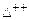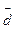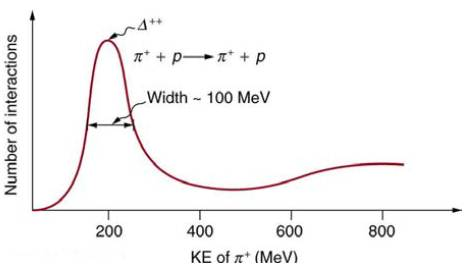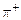Chapter 33, Problem 22PE

Chapter
Section
Textbook Problem

Accelerators such as the Triangle Universities Meson Facility (TRIUMF) in British Columbia produce secondary beams of pions by having an intense primary proton beam strike a target. Such “meson factories“ have been used for many years to study the interaction of pions with nuclei and, hence, the strong nuclear force. One reaction that occurs iswhere theis every short−lived particle. The graph in Figure 33.26 shows the probability of this reaction as a function of energy. The width of the bump is the uncertainty in energy due to the short lifetime at the(a) Find this lifetime.(b) Verify from the quark composition of the particles that this reaction annihilates and then re−creates a d quark and aantiquark by writing the reaction and decay in terms of quarks.(c) Draw a Feynman diagram of the production and decay of theshowing the individual quarks involved.Figure 33.26 This graph shows the probability of an interaction between aand a proton as a function of energy. The bump is interpreted as a very short lived particle called aThe approximately 100−MeV width of the bump is due to the short lifetime of theTo determine

(a)

The life time of Δ++ particle created at TRIUMF.

Explanation

Given:

Uncertainty in energy =100 MeV

Formula used:

The uncertainty principle for energy and time is given by, ΔE.Δt=h4π

Where, ΔE uncertainty in the measurement of energy

Δt uncertainty in the measurement of time

Calculation:

Therefore, uncertainty in short life time of Δ+ particle Δt=h4πΔE

=6

To determine

(b)

Whether the quark composition of this reaction annihilates and then recreates a d quark

and a d¯ antiquark

To determine

(c)

To draw Feynman diagram for the production and decay of Δ++ particle

Still sussing out bartleby?

Check out a sample textbook solution.

See a sample solution

The Solution to Your Study Problems

Bartleby provides explanations to thousands of textbook problems written by our experts, many with advanced degrees!

Get Started